JEE  >  Previous Year Questions: Laws of Motion - 2

# Previous Year Questions: Laws of Motion - 2

Test Description

## 9 Questions MCQ Test Physics Class 11 | Previous Year Questions: Laws of Motion - 2

Previous Year Questions: Laws of Motion - 2 for JEE 2023 is part of Physics Class 11 preparation. The Previous Year Questions: Laws of Motion - 2 questions and answers have been prepared according to the JEE exam syllabus.The Previous Year Questions: Laws of Motion - 2 MCQs are made for JEE 2023 Exam. Find important definitions, questions, notes, meanings, examples, exercises, MCQs and online tests for Previous Year Questions: Laws of Motion - 2 below.
Solutions of Previous Year Questions: Laws of Motion - 2 questions in English are available as part of our Physics Class 11 for JEE & Previous Year Questions: Laws of Motion - 2 solutions in Hindi for Physics Class 11 course. Download more important topics, notes, lectures and mock test series for JEE Exam by signing up for free. Attempt Previous Year Questions: Laws of Motion - 2 | 9 questions in 10 minutes | Mock test for JEE preparation | Free important questions MCQ to study Physics Class 11 for JEE Exam | Download free PDF with solutions
 1 Crore+ students have signed up on EduRev. Have you?
Previous Year Questions: Laws of Motion - 2 - Question 1

### Mass of the object is quantitative measure of its inertia stated law is newton's

Detailed Solution for Previous Year Questions: Laws of Motion - 2 - Question 1

Mass of the object is quantitative measure of its inertia stated law is Newton's First Law.

Previous Year Questions: Laws of Motion - 2 - Question 2

### Two blocks A and B masses 2m and m, respectively, are connected by a massless and inextensible string. The whole system is suspended by a massless spring as shown in the figure. The magnitudes of acceleration of A and B, immediately after the string is cut, are respectively.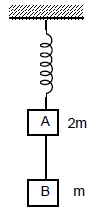[JEE 2006]

Detailed Solution for Previous Year Questions: Laws of Motion - 2 - Question 2

Before the string is cut the force applied by the spring is 3mg and tension in string is mg
Once the spring is cut, the tension comes to zero suddenly,
Thus acceleration of block b gets g downwards,
Whereas acceleration of a becomes g/2 upwards because the force from the spring is still the same at the moment the string is cut.

Previous Year Questions: Laws of Motion - 2 - Question 3

### A circular disc with a groove along its diameter is placed horizontally. A block of mass 1 kg is placed as shown. The co-efficient of friction between the block and all surfaces of groove in contact is m = 2/5. The disc has an acceleration of 25 m/s2. Find the acceleration of the block with respect to disc.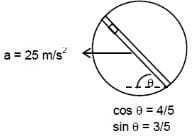[JEE 2006]

Detailed Solution for Previous Year Questions: Laws of Motion - 2 - Question 3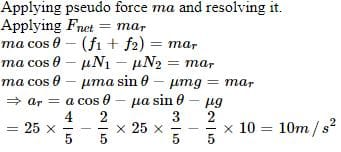Previous Year Questions: Laws of Motion - 2 - Question 4

STATEMENT-1 A cloth Covers a table. Some dishes are kept on it. The cloth can be pulled out without dislodging the dishes from the table

because

STATEMENT-2 For every action there is an equal and opposite reaction

Detailed Solution for Previous Year Questions: Laws of Motion - 2 - Question 4

“A cloth covers a table. Some dishes are kept on it. The cloth can be pulled out without dislodging the dishes from the table.” This is a very common phenomenon and can be seen when the cloth is pulled very quickly. But it can’t be explained by the 3rd Law of Motion.

Previous Year Questions: Laws of Motion - 2 - Question 5

Statement-1 It is easier to pull a heavy object than to push it on a level ground.

and

Statement-2 The magnitude of frictional force depends on the nature of the two surfaces in contact .

[ JEE 2008]

Detailed Solution for Previous Year Questions: Laws of Motion - 2 - Question 5

Both statements are independently true but 2 is not the correct explanation of 1.
The reason for statement 1 is that while pulling, a component of force reduces the normal reaction and hence reduces the friction force.

Previous Year Questions: Laws of Motion - 2 - Question 6

A block of base 10 cm × 10 cm and height 15 cm is kept on an inclined plane. The coefficient of friction between them is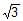. The inclination q of this inclined plane from the horizontal plane is gradually increased from 0º. Then                                                                                                 [jee 2009]

Detailed Solution for Previous Year Questions: Laws of Motion - 2 - Question 6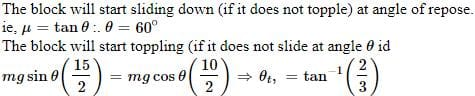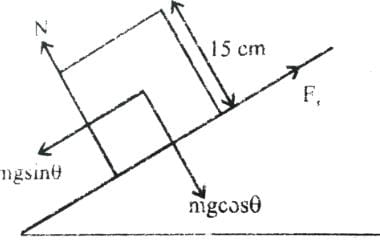Previous Year Questions: Laws of Motion - 2 - Question 7

A block of mass m is on an inclined plane of angle q. The coefficient of friction betwen the block and the plane is m and tan q > m. The block is held stationary by applying a force P parallel to the plane. The direction of force pointing up the plane is taken to the positive. As P is varied from P = mg (sin q _ m cos q ) to Pz = mg (sin q + m cos q), the frictional force f versus P graph will look like              [jee 2010]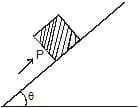Detailed Solution for Previous Year Questions: Laws of Motion - 2 - Question 7

f= sin Q−P

arry lineary
fi=mg sinθ−mg(sinθ − Hcosθ)
Hmg cosθ
1​=mgsinθ−mg(sinθ + Hcosθ)f
=−Hnmg sinθ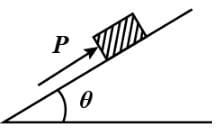Previous Year Questions: Laws of Motion - 2 - Question 8

A block is moving on an inclined plane making an angle 45º with horizontal and the coefficient of friciton is μ. the force required to just push it up the inclined plane is 3 times the force requried to just prevent it from sliding down. If we define N = 10μ, then N is

[jee 2011]

Detailed Solution for Previous Year Questions: Laws of Motion - 2 - Question 8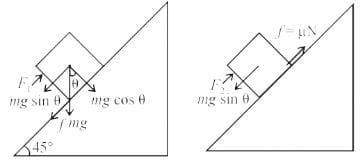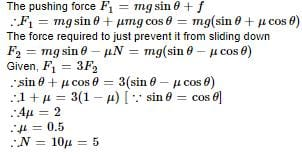*Multiple options can be correct
Previous Year Questions: Laws of Motion - 2 - Question 9

A small block of mass of 0.1 kg lies on a fixed inclined plane PQ which makes an angle with the horizontal. A horizontal force of 1 N acts on the block through its center of mass as shown in the figure. The block remains stationary if (take g = 10 m/s2)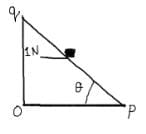Detailed Solution for Previous Year Questions: Laws of Motion - 2 - Question 9

Solution :- At θ=45∘,mgsinθ=1×cosθ

At θ>45∘,mgsinθ=1×cosθ (friction acts upward)

At θ<45∘,mgsinθ=1×cosθ (Friction acts downward)

xa=gsinθ−μgcosθ

## Physics Class 11

127 videos|464 docs|210 tests
 Use Code STAYHOME200 and get INR 200 additional OFF Use Coupon Code
Information about Previous Year Questions: Laws of Motion - 2 Page
In this test you can find the Exam questions for Previous Year Questions: Laws of Motion - 2 solved & explained in the simplest way possible. Besides giving Questions and answers for Previous Year Questions: Laws of Motion - 2, EduRev gives you an ample number of Online tests for practice

## Physics Class 11

127 videos|464 docs|210 tests